Quadruple Nested SUMX or CROSSJOIN - Excelerator BI

# Quadruple Nested SUMX or CROSSJOIN

Level: Intermediate

I had an interesting problem with a client last week where I built a data model to calculate rebates payable to customers based on their sales turnover.  There were a few challenges that I am going to cover below, including:

1. There needs to be a user friendly way to capture the rebate data, but it then needs to be transformed so it can be used in the model.
2. There is a nested SUMX problem – quadruple nesting in this case.  I solve this with a CROSSJOIN (and then SUMMARIZE) that I will explain below.
3. There is also a quadruple nested IF(HASONEVALUE()) problem that I solve with an innovative formula that I will also cover below.

The scenario is a wholesale business that sells products to customers.  Depending on the contract with the customer, a rebate may be paid on parts of the sale.  The objective is to create a tool that will correctly calculate the rebate payable at all aggregation levels in a pivot table.

## The Data Model

To demonstrate the problem I have built a simplified model that illustrates the problem and solutions.  It is worth noting that this is a simplified model – in reality the situation was more complex at various levels.  I have not brought those complexities into this post as doing so would push the difficulty up to “advanced” and that would miss the “intermediate” DAX learning opportunity.  I have uploaded my Demo Workbook here if you want to take a look.

### The 4 Levels of Granularity

The complexity of this problem comes from the 4 levels of granularity (there were more levels in the real problem but these 4 demonstrate the problem well).   The table below illustrates this granularity.  The requirement is to pay each customer a % rebate on sales turnover.  The customer rebate varies by division, supplier and product category.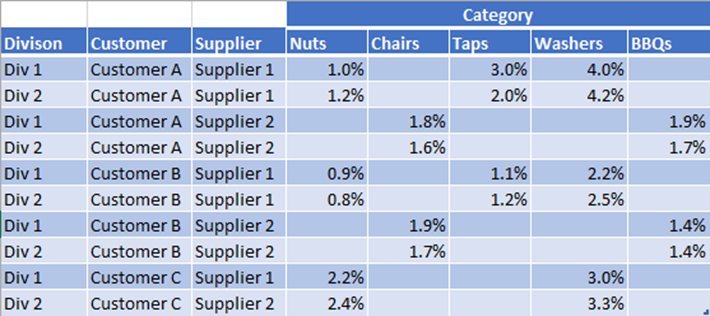The table above demonstrates a user friendly way to capture the rebate data from the user.  The table has category as columns – great for data entry but not so good for Power Pivot.  I then used Power Query to unpivot this data so it looks like this.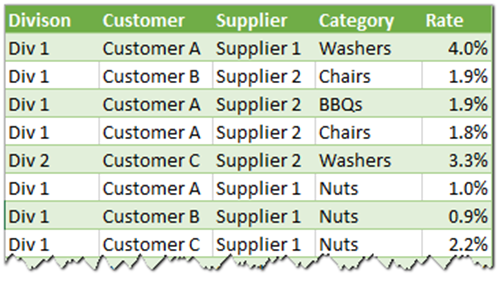The above table is much better for Power Pivot – a single column for each of the 4 dimensions (Division, Customer, Supplier, Category) and a single column that has the rebate rate.

### The Sales Table

The sales table was pretty straight forward.  I built a table that is in the same structure as the final rebate table above from the sales data warehouse as shown below.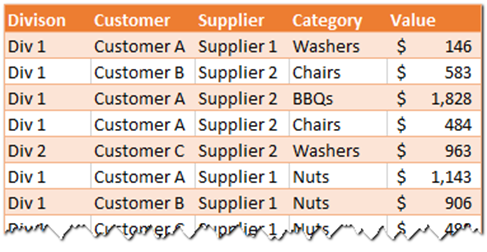I used the SQL for Excel Users techniques I covered in this blog post to shape the table on load, as the data warehouse did not have this summary level data natively stored.

### The Lookup Tables

I then needed 4 Lookup tables so that I could join both of the data tables and have them work together.  I used the technique covered in this blog post here to join the 2 data tables to the lookup tables and ended up with this.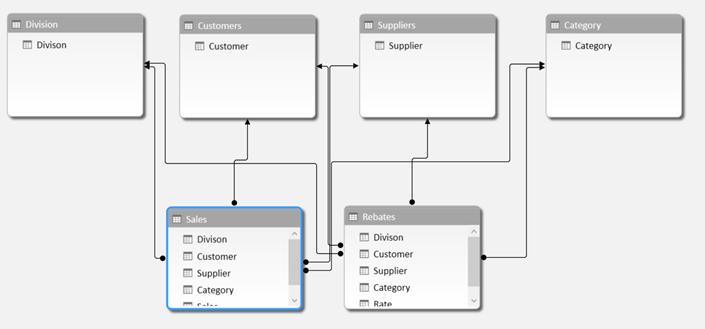Keep in mind this is a simplified data model to demonstrate some concepts.  If the real life example was the same as this, it would be possible (easy even) to combine the 2 data tables together into a single table in Power Query and simplify the problem.  But that would miss the opportunity to learn some more complex DAX, and the other complexities from the real scenario prevent this being a viable option anyway.

## The Sales Measure is Easy

The calculation of the Total Sales value is easy.

`Total Sales = SUM(Sales[Value])`

What’s more, this measure is fully additive.  So it doesn’t matter what level of granularity you have in the pivot table, the totals will always correctly roll up/down to reflect the correct totals at all levels as can be seen in the pivot table below.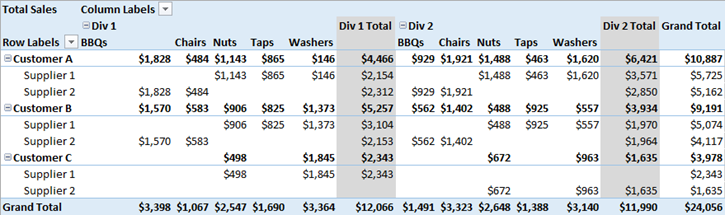## The Rebate Harvesting Measure

The rebate table is more complex.  The first thing to do is to “harvest” the correct rebate rate from the rebate table so it can be multiplied by the sales in the table above.  The raw measure seems pretty basic in the first instance.

`Rebate Rate = SUM(Rebates[Rate])`

This formula uses the SUM function as a trick to “harvest” the value from the table (given you can’t use a naked column inside a measure like this). It is possible to use any aggregation measure (I could have used MIN, MAX, AVERAGE or even VALUES) to do this.  I selected SUM in this instance to easily illustrate the aggregation problem – if I had used one of the others the problem would be much harder to spot.

Unlike the Sales table above (which is fully additive), these rebate rates are not additive at allThey only make sense at the level of granularity of Division, Category, Supplier and Customer – there is no way to correctly aggregate them at this time (we could do a weighted average later but that is out of scope).

So you can see in the pivot table below that the Grand Total Rows and Columns are incorrectly adding these rates together.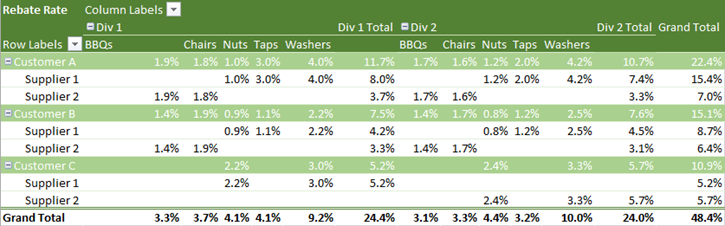As you can see in the pivot table above, the sub totals and grand totals incorrectly add the rebate rates and provide incorrect values at the aggregated levels. I wanted to force the pivot table to only return a value at the correct granularity (and return blanks otherwise to save confusion).  The way I normally do this is to use a IF(HASONEVALUE()) pattern.  This pattern works well on a single dimension, but in this case I would need to nest 4 of these patterns inside each other to correctly prevent the aggregations occurring in all instances. The formula would look like this.

```= IF(
HASONEVALUE(Division[Divison]),
IF(
HASONEVALUE(Suppliers[Supplier]),
IF(
HASONEVALUE(Customers[Customer]),
IF(HASONEVALUE(Category[Category]), SUM(Rebates[Rate]))
)
)
)```

As I thought through the issue of what I was actually trying to do, I came up with an innovative solution that was less complex to understand. I realised that the rebate rate only made sense if there was only 1 value for all of the dimension tables.  So I simply multiplied the number of rows visible in the filter context for each table by each other.  If the answer is 1, then the rebate rate is valid.  So the new formula I created was this.

```= IF(
COUNTROWS(Division) * COUNTROWS(Suppliers)
* COUNTROWS(Customers)
* COUNTROWS(Category) = 1,
SUM(Rebates[Rate])
)```

edit: late 16 March .  I have been thinking  I guess I could do something like this – haven’t tested it, but DAX formatter seems to like it!

```= IF(
HASONEVALUE(Division[Divison]) &&
HASONEVALUE(Suppliers[Supplier]) &&
HASONEVALUE(Customers[Customer]) && HASONEVALUE(Category[Category]), SUM(Rebates[Rate])
)```

The pivot table then updates and no longer returns incorrect values at the aggregate levels as shown below.  This makes it easy to see the rebate rate in any pivot table and it doesn’t show any incorrect aggregation values.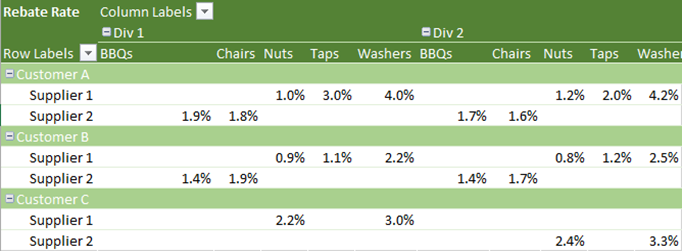## Time to Multiply the Measures

The next step is to multiply the Total Sales by the Rebate Rate to work out the amount payable.  I wrote a simple measure as follows and then got the pivot table below (yellow).

`Total Rebate Payable Wrong = [Total Sales] * [Rebate Rate]`

Note how there are no sub totals or grand totals – not what I want.  The problem is that the filtering I applied in the [Rebate Rate] measure above is filtering out the aggregated totals.  This filtering is good to prevent the pivot table displaying incorrect aggregations of the rate in the green table above, but it is causing a problem here.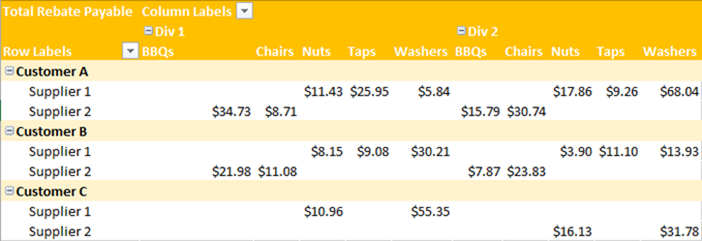So the next step I did was to modify the Total Rebate Payable measure as follows (removing the aggregation filter and just using the original raw measure).

`Total Rebate Payable Wrong = [Total Sales] * SUM(Rebates[Rate])`

## Now for the Problem and Purpose of this Post

This new measure is now creating sub totals and grand totals as shown below.  But the problem is that these totals are not correct.  Take the column of data highlighted shown below.  If you add up the rebates for Customer A, Supplier 2 = \$34.73 and Customer B, Supplier 2 = \$21.98 then you get \$56.71.  But the total of the column reads \$112.13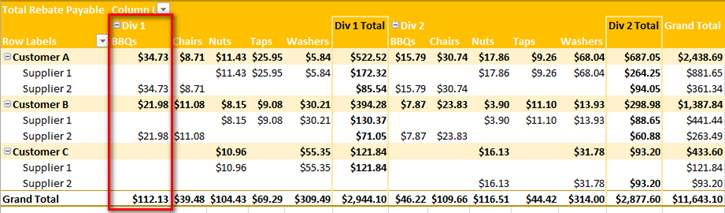The value in the grand total of this column is actually (Customer A, Supplier 2 Rebate + Customer B, Supplier 2 Rebate 3.3%) * (Combined Sales for both Customers \$3,398) = \$112.13.  This is simply the way Power Pivot and Pivot Tables work, so don’t fight it but learn to work with it.  As soon as you remove one of the filters from the pivot table (as happens automatically with sub totals and grand totals) then the whole thing falls apart.  This is now the classic problem that is normally solved by SUMX or by applying filters on ALL DIMENSIONS in the Pivot Table.

## The Quadruple Nested SUMX Solution

### Simplified SUMX

SUMX solves these problems by iterating over all the possible values in a table (or column turned into a table with VALUES) and does the calculation at each level of granularity.  For example, if we take a simplified example where there is just “customers” (ie no Suppliers, Categories, Divisions), the following formula would correctly calculate the rebate at the granular level as well as the totals.

```Simple SUMX Solution =
SUMX(Customers, [Total Sales] * CALCULATE(SUM(Rebates[Rebate])))```

The above formula iterates over the Customers table.  The [Total Sales] measure has an implicit CALCULATE wrapped around it, and I have included a CALCULATE around the Rebates[Rate] portion of the formula.  These 2  CALCULATES force context transition from the row context (created by SUMX) in the customers table into a filter context that filters both the Sales table and the Rebates table.  The net result is a formula that calculates the correct answer at the customer level regardless of the level of granularity in the pivot table (for this simplified example anyway).

But of course this simplified example only solves the granularity problem on one of the dimensions, and I have 4 in this example.  So one way to solve the problem is to nest 4 SUMX formulas together as follows:

```Total Rebate Payable SUMX =
SUMX(Customers,
SUMX(Category,
SUMX(Suppliers,
SUMX(Division, [Total Sales] * CALCULATE(SUM(Rebates[Rate])))
)
)
)
```

This formula solves the problems and gives the right answers as can be seen in the pivot table below. I like to think of SUMX as “simulating” the filtering behaviour of a pivot table in memory so that it always calculates the correct result.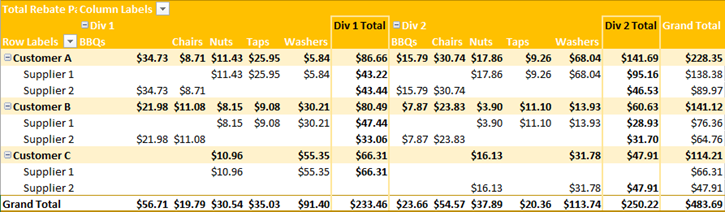But there are lots of problems with this formula, not least of which is that it is very inefficient.  This is not a problem on small data models but is a problem when you get more data.  Plus the formula is plain ugly.

## Enter CROSSJOIN

Now it is time to show you the CROSSJOIN alternative formula (which also works).  There is an even better formula at the end of the post.

```Total Rebate Payable =
SUMX(
CROSSJOIN(Customers, Category, Suppliers, Division),
[Total Sales] * CALCULATE(SUM(Rebates[Rate]))
)
```

This formula also uses a SUMX, but this time there is only a single SUMX function.  SUMX will iterate over a temporary table created by CROSSJOIN.  The temporary table contains a list of all the possible combinations of the 4 tables – exactly what we want to do to get the lowest level of granularity.  The easiest way to show this is to fire up DAX Studio and build the table.  See the results below.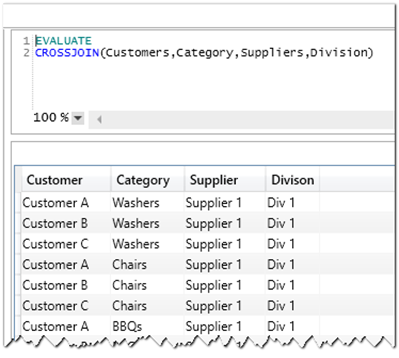This table has 60 rows in total – all of the possible combinations.  When the CROSSJOIN table is used inside the SUMX formula, a very important thing happens – this new temporary table retains a relationship to the data model.  So as SUMX iterates over this table, context transition will force the entire data model to be filtered at each step of the iteration of the temporary table.

I like to “imagine” a new table morphing at the top of the data model like illustrated below (this is not real, just an illustration of what I imagine).  I find it helpful to imagine it like this as I can then imagine the filters flowing down hill from the tables at the top to the tables at the bottom.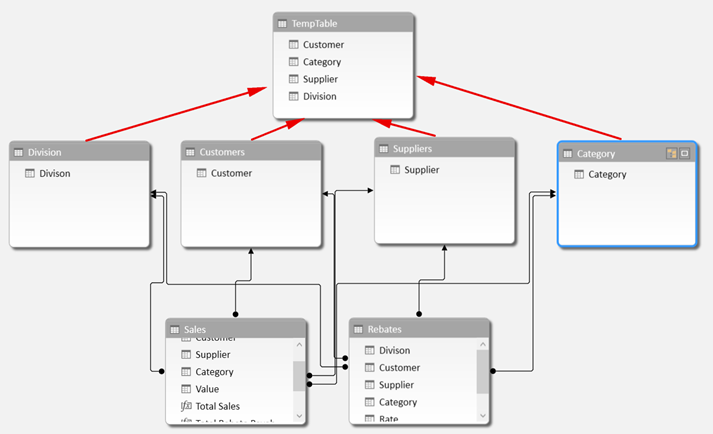CROSSJOIN always takes tables as inputs.  So it joins 2 or more tables together to create every combination.  But if you want to create a combination of the unique values in 2 columns in 2 different tables, you can use VALUES for that (example shown below).

```= SUMX(
CROSSJOIN(VALUES(Table1[Column A]), VALUES(Table2[Column B])),
[Measure 1] * [Measure 2]
)```

## An Even Better Solution using SUMMARIZE

Finally, there is actually a better solution than the CROSSJOIN solution above – the better solution uses SUMMARIZE.  The main difference between SUMMARIZE and CROSSJOIN (in this example anyway) is that SUMMARIZE will only return rows for valid combinations in the data model.  I put the SUMMARIZE table function in DAX Studio and it returned 24 rows (vs 60 rows in the CROSSJOIN).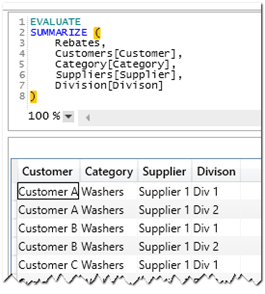With this knowledge, the more efficient formula using SUMMARIZE would be as follows, and the SUMX will only need to iterate the combinations that actually exist in the Rebate table.

```Total Rebate Payable =
SUMX(
SUMMARIZE(
Rebates,
Customers[Customer],
Category[Category],
Suppliers[Supplier],
Division[Divison]
),
[Total Sales] * CALCULATE(SUM(Rebates[Rate]))
)
```

Hopefully this has helped demystify SUMX, CROSSJOIN and SUMMARIZE (partially at least) and helped broaden your understanding of DAX.

### 9 thoughts on “Quadruple Nested SUMX or CROSSJOIN”

1.Dear Mr. Allington,

Thank you so much for this post, I was stuck with similar issue for couple of days and this helped me move to a next stage. The next stage in my case is year-to-date value. Could you give me any advice on how to get correct subtotals and grand totals in year-to-date calculation of a value similar by nature to total rebates?
I figured out that the problem is in rebate harvesting measure – when I calculate DATESYTD, the total I get is a sum of all rebates times total sales. Is there any way to work around this and get a correct total?
Thank you so much.

1.It’s hard to say with this level of information. I suggest for this one that you ask a question at community.powerbi.com providing as much detail as you can.

2.I am trying to use this pattern but need to filter my data so the equivalent measure for [Total Sales] looks like CALCULATE(SUM ( Sales[Value] ), [xxx]=1) this seems to throw things right out and give me wrong numbers. Any ideas on why this does not work based on using the SUMX and SUMMARIZE pastern. Thanks Matt.

1.With this pattern, [xxx] would force context transition within each iteration of sumx before the rest of CALCULATE(sum[sales[value],[xxx]=1) is calculated. My guess is this is not what you want. If you need [xxx] to be evaluated outside of the sumx row context, you could use the var/return syntax to evaluate [xxx] before entering the iteration. Alternatively you could try replacing the measure [xxx] with the raw formula. But these are just guesses from me based on what I can conceive “could” go wrong. I would need to see it to be sure. But give these things a try

3.Great post! I like the hasonevalue alternative!

However, I do find that using Crossjoin can be faster than summarize depending on the cardinality of your temp table. I often find that performance can be boosted between 10 – 50% by experimenting with the two.

I believe summarize will trigger an expensive table scan whereas Crossjoin does not.

1.Hi Simon,

in Matt’s example CROSSJOIN actually generates table scans (4 of them) . In order to avoid table scans you would have to do this – as I understand it:

Total Rebate Payable =
SUMX (
CROSSJOIN ( ALL(Customers), ALL(Category), ALL(Suppliers), ALL(Division) ),
[Total Sales] * CALCULATE ( SUM ( Rebates[Rate] ) )
)

By surrounding the tables with ALL, instead of scanning the tables used in CROSSJOIN the values from the dictionaries of the columns involved are used. Whether this is an advantage depends on the distribution of the data (granularities) and how much of the alternatives will be cached when you look at the pivot table as a whole. It’s actually quite tricky to figure that out beforehand as you have discovered yourself.

SUMMARIZE always returns exactly the number of existing combinations for a given filter context. This means that there are no superfluous iterations in SUMX whereas CROSSJOIN potentially could generate many superfluous iterations. The downside of SUMMARIZE is that in principle it can be impossible to cache and reuse a result from one cell to the next. (In principle because the engine is so damned clever that even that is difficult to judge). In my example with the CROSSJOIN with ALLs the SUMX will have to iterate through all possible combinations of Customer, Category, Supplier and Division many of which do not exist, however there are no table scans involved. In Matt’s original example the tables in CROSSJOIN are filtered by the filter context of the pivot table and hence will only generate more than one row (iteration) in subtotals and the grand total. Of course some of the combinations will not exist but SUMX will not have to iterate too many times in vain AND some of the table scans in CROSSJOIN will be cached from one cell to the next.

This is at least my take of it.

PS: An extension to Excel called ‘Olap Pivot Table Extention’ exists here http://olappivottableextend.codeplex.com. By using this extension you can get the MDX code used by excel to query the data model, then paste that code into DAX studio and run it from there and get an overview of how the entire pivot table is performing. That very often makes a difference compared to just testing a single dax measure in DAX studio. (Because of caching, filter context etc.)

4.Great post!

However, I do find that using Crossjoin can be faster than summarize depending on the cardinality of your temp table. I often find that performance can be boosted between 10 – 50% by experimenting with the two.

I believe summarize will trigger an expensive table scan whereas Crossjoin does not.

5.Matt, this is an awesome blog post. Thank you for thinking through this and coming up with an elegant solution.

1.You should try spending a week reading and learning from 1) The Definitive Guide to DAX and 2) a week of live training with Marco Russo – then anything is possible 🙂

Scroll to Top